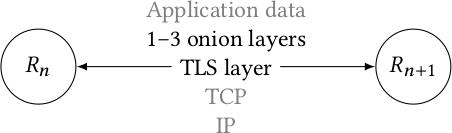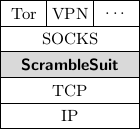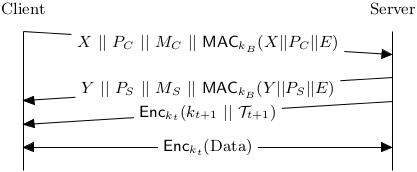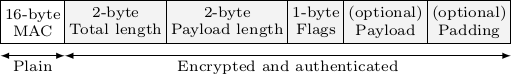Tikz Examples

# TikZ examples #

## Tor protocol stack #\tikzset{>=latex}
\begin{tikzpicture}

\node[circle,draw,minimum size=1cm] (R1) at (0, 0) {$R_n$};
\node[circle,draw,minimum size=1cm] (R2) at (5, 0) {$R_{n+1}$};

\draw[<->] (R1.east) -- node [midway, fill=white] (line) {\phantom{TLS layer}} (R2.west);

\node[align=center] at (line) {{\color{gray} Application data}\\1--3 onion
layers\\TLS layer\\{\color{gray}TCP}\\{\color{gray} IP}};

\end{tikzpicture}


## Tor’s hash ring #% Change arrow head globally.
\tikzset{>=latex}
\begin{tikzpicture}

\draw[,->] (0,0) node[anchor=north] {\texttt{0x00..00}} -- (6.8,0) node[anchor=north] {\texttt{0xff..ff}};

\draw[fill=white] (0.1,0) circle (1mm);
\draw[fill=white] (0.6,0) circle (1mm);
\draw[fill=white] (1,0) circle (1mm);
\draw[fill=white] (1.5,0) circle (1mm);
\draw[fill=white] (1.7,0) circle (1mm);

\draw[fill=red] (2,0) circle (1mm);

\draw[,->] (2,0) -- (1.7,-0.5) node[anchor=north] {Onion service};

\draw[fill=cyan] (2.3,0) circle (1mm);
\draw[fill=cyan] (2.7,0) circle (1mm);
\draw[fill=cyan] (3,0) circle (1mm);
\draw[,->] (2.3,0) -- (2.6,0.5);
\draw[,->] (2.7,0) -- (2.7,0.5) node[anchor=south] {HSDir replica \#1};
\draw[,->] (3.0,0) -- (2.8,0.5);

\draw[fill=white] (3.3,0) circle (1mm);
\draw[fill=white] (3.6,0) circle (1mm);

\draw[fill=orange] (4,0) circle (1mm);
\draw[fill=orange] (4.2,0) circle (1mm);
\draw[fill=orange] (4.6,0) circle (1mm);
\draw[,->] (4.0,0) -- (4.4,-0.5);
\draw[,->] (4.2,0) -- (4.5,-0.5) node[anchor=north] {HSDir replica \#2};
\draw[,->] (4.6,0) -- (4.6,-0.5);

\draw[fill=white] (5,0) circle (1mm);
\draw[fill=white] (5.3,0) circle (1mm);
\draw[fill=white] (5.8,0) circle (1mm);
\draw[fill=white] (6.2,0) circle (1mm);
\draw[fill=white] (6.5,0) circle (1mm);
\draw[,->] (5.0,0) -- (5.6,0.5);
\draw[,->] (5.3,0) -- (5.7,0.5);
\draw[,->] (5.8,0) -- (5.8,0.5) node[anchor=south] {HSDirs};
\draw[,->] (6.2,0) -- (5.9,0.5);
\draw[,->] (6.5,0) -- (6.0,0.5);

\end{tikzpicture}


## Network protocol stack #\begin{tikzpicture}[node distance=0 cm,outer sep = 0pt,inner sep = 2pt]
\tikzset{field/.style={align=center,shape=rectangle,minimum height=0.5cm,minimum width=9mm,draw}}
\tikzset{largefield/.style={align=center,shape=rectangle,minimum height=0.5cm,minimum width=27mm,draw}}

\node [field] (tor) {Tor};
\node [field,right=of tor] (vpn) {VPN};
\node [field,right=of vpn] {\dots};

\node [largefield,below=of vpn] (socks) {SOCKS};
\node [largefield,below=of socks,fill=gray!30] (scramblesuit) {\textbf{\pt{}}};
\node [largefield,below=of scramblesuit] (tcp) {TCP};
\node [largefield,below=of tcp] {IP};
\end{tikzpicture}


## Network communication #\begin{tikzpicture}
\draw (0,0) -- (0,3);
\draw (8,0) -- (8,3);

\draw [arrows={-triangle 45}]
(0, 3) -- (8, 2.5) node [midway, fill=white, text centered]
{ $X\ ||\ P_{C}\ ||\ M_{C}\ ||\ \textsf{MAC}_{k_{B}}(X||P_{C}||E)$ };

\draw [arrows={triangle 45-}]
(0, 1.5) -- (8, 2) node [midway, fill=white, text centered]
{ $Y\ ||\ P_{S}\ ||\ M_{S}\ ||\ \textsf{MAC}_{k_{B}}(Y||P_{S}||E)$ };

\draw [arrows={-triangle 45}]
(8, 1.5) -- node [midway, fill=white, text centered]
{ $\textsf{Enc}_{k_{t}}(k_{t+1}\ ||\ \mathcal{T}_{t+1})$ } (0, 1);

\draw [arrows={triangle 45-triangle 45}]
(0, 0.5) -- node [midway, fill=white]
{ $\textsf{Enc}_{k_{t}}$(Data) } (8, 0.5);

\node at (0,3.5) {Client};
\node at (8,3.5) {Server};
\end{tikzpicture}\begin{tikzpicture}[node distance=0 cm,outer sep = 0pt,
inner sep = 2pt,font=\scriptsize]
\tikzset{field/.style={align=center,shape=rectangle,
minimum height=0.7cm,draw}}
\tikzset{darkfield/.style={fill=gray!10,align=center,shape=rectangle,
minimum height=0.7cm,draw}}

\node [field]                      (mac)     {16-byte\\MAC};
\node [darkfield,right=of mac]     (tlen)    {2-byte\\Total length};
\node [darkfield,right=of tlen]    (plen)    {2-byte\\Payload length};
\node [darkfield,right=of plen]    (flags)   {1-byte\\Flags};
\draw [latex-latex] ($(mac.south west) - (0,0.2)$) -- node [auto,swap]
{Plain} ($(mac.south east) - (0,0.2)$);
\draw [latex-latex] ($(tlen.south west) - (0,0.2)$) -- node [auto,swap]
{Encrypted and authenticated} ($(padding.south east) - (0,0.2)$);